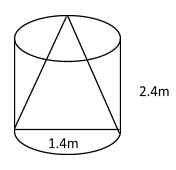Courses
Courses for Kids
Free study material
Offline Centres
MoreLast updated date: 23rd Nov 2023
Total views: 279.9k
Views today: 6.79k

# From the solid cylinder whose height is $2.4cm$ and diameter $1.4cm$, a conical cavity of the same height and same diameter is hollowed out. Find the volume of the remaining solid to the nearest cm cube. Find its total surface area.Verified
279.9k+ views
Hint: The area can be defined as the space occupied by a flat surface of an object. The area is the number of unit squares closed by figure. Perimeter is the total length of the sides of the two dimensional shape. Perimeter is always less than the area of the given figure. Because the perimeter is outer and the area is inner property. Volume is the capacity which hold by objects
As we know that
$\therefore V = \dfrac{1}{3}\pi {r^2}h$
Here
V=volume
h=height

Complete step-by-step solution:
Given,Diameter of cylinder,$d = 1.4cm$
Radius of cylinder, $r = \dfrac{{1.4}}{2}cm$
Radius of cylinder, $r = 0.7cm$
Height of cylinder, $h = 2.4cm$
Diameter of cone,$d = 1.4cm$
Radius of cone, $r = \dfrac{{1.4}}{2}cm$
Radius of cone, $r = 0.7cm$
Height of cone, $h = 2.4cm$
Volume=?
Now the volume of cylinder
As we know that
$\therefore V = \pi {r^2}h$
Put the value
$\Rightarrow V = \dfrac{{22}}{7} \times {(0.7)^2} \times 2.4$
Simplify
$\Rightarrow V = 22 \times 0.7 \times 2.4$
$\Rightarrow {V_{cylinder}} = 36.96c{m^3}$
Now volume of cone
$\therefore V = \dfrac{1}{3}\pi {r^2}h$
Put the value
$\Rightarrow V = \dfrac{1}{3} \times \dfrac{{22}}{7} \times {(0.7)^2} \times 2.4$
Simplify
$\Rightarrow V = 22 \times 0.7 \times 0.8$
$\Rightarrow {V_{cone}} = 12.32c{m^3}$
Volume remaining
$\Rightarrow {V_{remaining}} = {V_{cylinder}} - {V_{cone}}$
Put the value
$\Rightarrow {V_{remaining}} = 36.96 - 12.32$
$\Rightarrow {V_{remaining}} = 24.64c{m^3}$
Now the total curved surface area of cylinder
As we know that
$\therefore 2\pi rh$
Put the value
$\Rightarrow {A_{cylinder}} = 2 \times \dfrac{{22}}{7} \times 0.7 \times 2.4$
Simplify
$\Rightarrow {A_{cylinder}} = 44 \times 0.1 \times 2.4$
$\Rightarrow {A_{cylinder}} = 10.56c{m^2}$
Now curved surface area of cone,
$\therefore {A_{cone}} = \pi rl$
Now find the slant height l=
$\therefore l = \sqrt {{r^2} + {h^2}}$
Put the value
$\Rightarrow l = \sqrt {{{(0.7)}^2} + {{(2.4)}^2}}$
$\Rightarrow l = \sqrt {0.49 + 5.76}$
$\Rightarrow l = \sqrt {6.25}$
$\Rightarrow l = 2.5cm$
As we know that
$\therefore {A_{cone}} = \pi rl$
Put value
$\Rightarrow {A_{cone}} = \dfrac{{22}}{7} \times 0.7 \times 2.5$
$\Rightarrow {A_{cone}} = 2.2 \times 2.5$
$\Rightarrow {A_{cone}} = 5.5c{m^2}$
Area of base is given by
As we know that
$\therefore {A_c} = \pi {r^2}$
Put the value
$\Rightarrow {A_c} = \dfrac{{22}}{7} \times {(0.7)^2}$
Simplify
$\Rightarrow {A_c} = \dfrac{{22}}{7} \times 0.7 \times 0.7$
$\Rightarrow {A_c} = 2.2 \times 0.7$
$\Rightarrow {A_c} = 1.54c{m^2}$
Total curved surface area
$\therefore {A_T} = {A_{cylinder}} + {A_{cone}} + {A_{circle}}$
Put the value
$\Rightarrow {A_T} = 10.56 + 5.5 + 1.54$
$\Rightarrow {A_T} = 17.6c{m^2}$
Hence the total surface area of cylinder is$17.6c{m^2}$

Note: Curved surface area means it covered the area or curved surface. It leaves that area of base of top and bottom. When we talk about the total surface area the area of base and curved surface area will include. There is no parameter of 3-D geometry.